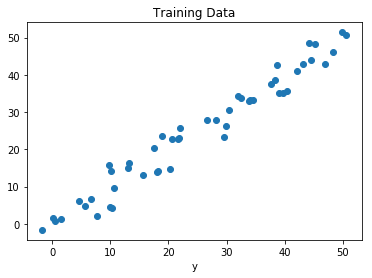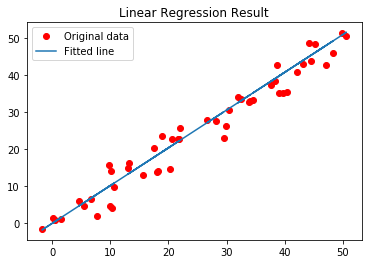# Linear Regression Using Tensorflow

## Prerequisites

We will briefly summarize Linear Regression before implementing it using Tensorflow. Since we will not get into the details of either Linear Regression or Tensorflow, please read the following articles for more details:

## Brief Summary of Linear Regression

Linear Regression is a very common statistical method that allows us to learn a function or relationship from a given set of continuous data. For example, we are given some data points of x and corresponding y and we need to learn the relationship between them that is called a hypothesis.

In case of Linear regression, the hypothesis is a straight line, i.e,Where w is a vector called Weights and b is a scalar called Bias. The Weights and Bias are called the parameters of the model.

All we need to do is estimate the value of w and b from the given set of data such that the resultant hypothesis produces the least cost J which is defined by the following cost functionwhere m is the number of data points in the given dataset. This cost function is also called Mean Squared Error.

For finding the optimized value of the parameters for which J is minimum, we will be using a commonly used optimizer algorithm called Gradient Descent. Following is the pseudo-code for Gradient Descent:

 Repeat untill Convergence { w = w - α * δJ/δw b = b - α * δJ/δb } 

where α is a hyperparameter called the Learning Rate.

## Tensorflow

Tensorflow is an open-source computation library made by Google. It is a popular choice for creating applications that require high-end numerical computations and/or need to utilize Graphics Processing Units for computation purposes. These are the main reasons due to which Tensorflow is one of the most popular choices for Machine Learning applications, especially Deep Learning. It also has APIs like Estimator which provide a high level of abstraction while building Machine Learning Applications. In this article, we will not be using any high-level APIs, rather we will be building the Linear Regression model using low-level Tensorflow in the Lazy Execution Mode during which Tensorflow creates a Directed Acyclic Graph or DAG which keeps track of all the computations, and then executes all the computations done inside a Tensorflow Session.

## Implementation

We will start by importing the necessary libraries. We will use Numpy along with Tensorflow for computations and Matplotlib for plotting.

 import numpy as np  import tensorflow as tf  import matplotlib.pyplot as plt

In order to make the random numbers predictable, we will define fixed seeds for both Numpy and Tensorflow.

 np.random.seed(101)  tf.set_random_seed(101)

Now, let us generate some random data for training the Linear Regression Model.

 # Genrating random linear data  # There will be 50 data points ranging from 0 to 50  x = np.linspace(0, 50, 50)  y = np.linspace(0, 50, 50)     # Adding noise to the random linear data  x += np.random.uniform(-4, 4, 50)  y += np.random.uniform(-4, 4, 50)     n = len(x) # Number of data points

Let us visualize the training data.

 # Plot of Training Data  plt.scatter(x, y)  plt.xlabel('x')  plt.xlabel('y')  plt.title("Training Data")  plt.show()

Output:Now we will start creating our model by defining the placeholders X and Y, so that we can feed our training examples X and Y into the optimizer during the training process.

 X = tf.placeholder("float")  Y = tf.placeholder("float")

Now we will declare two trainable Tensorflow Variables for the Weights and Bias and initializing them randomly using np.random.randn().

 W = tf.Variable(np.random.randn(), name = "W")  b = tf.Variable(np.random.randn(), name = "b")

Now we will define the hyperparameters of the model, the Learning Rate and the number of Epochs.

 learning_rate = 0.01 training_epochs = 1000

Now, we will be building the Hypothesis, the Cost Function, and the Optimizer. We won’t be implementing the Gradient Descent Optimizer manually since it is built inside Tensorflow. After that, we will be initializing the Variables.

 # Hypothesis  y_pred = tf.add(tf.multiply(X, W), b)     # Mean Squared Error Cost Function  cost = tf.reduce_sum(tf.pow(y_pred-Y, 2)) / (2 * n)     # Gradient Descent Optimizer  optimizer = tf.train.GradientDescentOptimizer(learning_rate).minimize(cost)     # Global Variables Initializer  init = tf.global_variables_initializer()

Now we will begin the training process inside a Tensorflow Session.

 # Starting the Tensorflow Session  with tf.Session() as sess:             # Initializing the Variables      sess.run(init)             # Iterating through all the epochs      for epoch in range(training_epochs):                     # Feeding each data point into the optimizer using Feed Dictionary          for (_x, _y) in zip(x, y):              sess.run(optimizer, feed_dict = {X : _x, Y : _y})                     # Displaying the result after every 50 epochs          if (epoch + 1) % 50 == 0:              # Calculating the cost a every epoch              c = sess.run(cost, feed_dict = {X : x, Y : y})              print("Epoch", (epoch + 1), ": cost =", c, "W =", sess.run(W), "b =", sess.run(b))             # Storing necessary values to be used outside the Session      training_cost = sess.run(cost, feed_dict ={X: x, Y: y})      weight = sess.run(W)      bias = sess.run(b)

Output:

Epoch: 50 cost = 5.8868036 W = 0.9951241 b = 1.2381054
Epoch: 100 cost = 5.7912707 W = 0.99812365 b = 1.0914398
Epoch: 150 cost = 5.7119675 W = 1.0008028 b = 0.96044314
Epoch: 200 cost = 5.6459413 W = 1.0031956 b = 0.8434396
Epoch: 250 cost = 5.590799 W = 1.0053328 b = 0.7389357
Epoch: 300 cost = 5.544608 W = 1.007242 b = 0.6455922
Epoch: 350 cost = 5.5057883 W = 1.008947 b = 0.56222
Epoch: 400 cost = 5.473066 W = 1.01047 b = 0.48775345
Epoch: 450 cost = 5.4453845 W = 1.0118302 b = 0.42124167
Epoch: 500 cost = 5.421903 W = 1.0130452 b = 0.36183488
Epoch: 550 cost = 5.4019217 W = 1.0141305 b = 0.30877414
Epoch: 600 cost = 5.3848577 W = 1.0150996 b = 0.26138115
Epoch: 650 cost = 5.370246 W = 1.0159653 b = 0.21905091
Epoch: 700 cost = 5.3576994 W = 1.0167387 b = 0.18124212
Epoch: 750 cost = 5.3468933 W = 1.0174294 b = 0.14747244
Epoch: 800 cost = 5.3375573 W = 1.0180461 b = 0.11730931
Epoch: 850 cost = 5.3294764 W = 1.0185971 b = 0.090368524
Epoch: 900 cost = 5.322459 W = 1.0190892 b = 0.0663058
Epoch: 950 cost = 5.3163586 W = 1.0195289 b = 0.044813324
Epoch: 1000 cost = 5.3110332 W = 1.0199214 b = 0.02561663

Now let us look at the result.

 # Calculating the predictions  predictions = weight * x + bias  print("Training cost =", training_cost, "Weight =", weight, "bias =", bias, '\n')

Output:

Training cost = 5.3110332 Weight = 1.0199214 bias = 0.02561663

Note that in this case both the Weight and bias are scalars. This is because, we have considered only one dependent variable in out training data. If we have m dependent variables in our training dataset, the Weight will be an m-dimensional vector while bias will be a scalar.

Finally, we will plot our result.

 # Plotting the Results  plt.plot(x, y, 'ro', label ='Original data')  plt.plot(x, predictions, label ='Fitted line')  plt.title('Linear Regression Result')  plt.legend()  plt.show()

Output:Attention geek! Strengthen your foundations with the Python Programming Foundation Course and learn the basics.

To begin with, your interview preparations Enhance your Data Structures concepts with the Python DS Course.

My Personal Notes arrow_drop_upI am a 3rd year student at School of Computer Engineering at Kalinga Institute of Industrial Technology My interests include Machine Learning Computer Vision Game Development Web Technologies Competitive Programming and Creative Programming

If you like GeeksforGeeks and would like to contribute, you can also write an article using contribute.geeksforgeeks.org or mail your article to contribute@geeksforgeeks.org. See your article appearing on the GeeksforGeeks main page and help other Geeks.

Please Improve this article if you find anything incorrect by clicking on the "Improve Article" button below.

Improved By : rakshitasinghal

Practice Tags :

4

Please write to us at contribute@geeksforgeeks.org to report any issue with the above content.